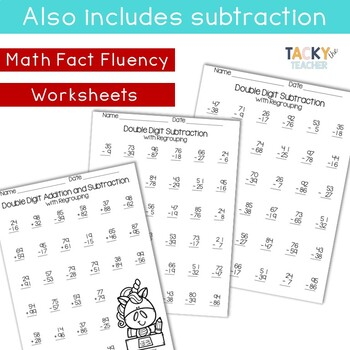# Unicorn Math Fluency Pack | Double Digit Addition & Subtraction with Regrouping

Rated 4.82 out of 5, based on 17 reviews
17 Ratings;
2nd - 3rd
Subjects
Resource Type
Standards
Formats Included
• PDF
\$1.60
List Price:
\$2.00
You Save:
\$0.40
\$1.60
List Price:
\$2.00
You Save:
\$0.40
Report this resource to TPT

#### What educators are saying

I used this for a student to practice fluency who was above addition to 20 and she loved these pages!
##### Also included in
1. This bundle has a variety of engaging unicorn activities to print (20 math and literacy pages, a Sentence Scramble writing activity, AND Addition and Subtraction Card Game including addition facts within 20 and subtraction fact within 10) with unicorn graphics! I created it because I needed a fun an
Price \$4.60Original Price \$8.25Save \$3.65

### Description

You are purchasing my BEST-SELLING math fact fluency pack for two digit addition and subtraction with regrouping (carrying and borrowing)! This pack has adorable unicorns, rainbows, and hearts perfect for any time of year.

I created it because I needed a fun and engaging way to practice math fact fluency with my students. My goal was to create a resource that was print and go!

What’s Included?

Two digit addition and subtraction facts with regrouping for:

• Adding 2 two digit numbers (2 pages)
• Adding 3 two digit numbers (1 page)
• Adding 4 two digit numbers (1 page)
• Subtracting 2 two digit numbers (2 pages)

★ Pick and choose which pages you want to do, print, and you're all set! You can time students for several minutes to see how many problems they can do, and use to inform instruction. Or they can complete it as a math center or during morning work.

Check out my other math resources available on TpT!

★ My Spring Math Fluency Pack has a variety of engaging activities (36 pages!) with absolutely NO PREP!

★ Try my Summer Math Fact Fluency for Multiplication, which has 14 pages of fact practice for students who are just beginning to learn multiplication (0, 1, 2, 5, & 10 facts)!

Would you like to have access to this and other teaching resources? Stop by and visit my blog for a peek!

Tacky the Teacher

Don't forget to follow my store for notification of new products and sales!

Thank you for all you do!

Ashley :)

Total Pages
N/A
Teaching Duration
N/A
Report this resource to TPT
Reported resources will be reviewed by our team. Report this resource to let us know if this resource violates TPT’s content guidelines.

### Standards

to see state-specific standards (only available in the US).
Apply properties of operations as strategies to add and subtract. If 8 + 3 = 11 is known, then 3 + 8 = 11 is also known. (Commutative property of addition.) To add 2 + 6 + 4, the second two numbers can be added to make a ten, so 2 + 6 + 4 = 2 + 10 = 12. (Associative property of addition.)
Add and subtract within 20, demonstrating fluency for addition and subtraction within 10. Use strategies such as counting on; making ten (e.g., 8 + 6 = 8 + 2 + 4 = 10 + 4 = 14); decomposing a number leading to a ten (e.g., 13 - 4 = 13 - 3 - 1 = 10 - 1 = 9); using the relationship between addition and subtraction (e.g., knowing that 8 + 4 = 12, one knows 12 - 8 = 4); and creating equivalent but easier or known sums (e.g., adding 6 + 7 by creating the known equivalent 6 + 6 + 1 = 12 + 1 = 13).
Fluently add and subtract within 20 using mental strategies. By end of Grade 2, know from memory all sums of two one-digit numbers.